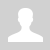Python. Tuples (Кортежи)

11.2.4.1 create a tuple;

Python. Tuples (Кортежи)

## We already know collections such as lists, sets, and strings.

Tuples are very similar to lists; they are also an indexed collection, only they use parentheses instead of square ones (and can often be omitted):

```# a tuple of two elements; element type can be any data type card = ('Amir', 5) # empty tuple (of 0 elements) empty = () # one element tuple - comma needed if t a tuple marks = (4,) # length, value of a single element, addition - like lists print (len(card), card, card + marks) -------------------------------------------------- Output: 2 5 ('Amir', 5, 4)```

Comparisons of tuples

Tuples can be compared with each other

```(1, 2, 3) == (1, 2, 3) # True (1, 2) == (1, 3) # False (1, 2) < (1, 3) # True (1, 2) < (5,) # True ('12', 'school') < ('12', 'class') # False # But you can't compare like this: elements of tuples of different types (1, 2) < ('5', 'Amir') # '<' not supported  between instances  of 'int' and 'str'```

Note that the operations == and !=  are applicable to any tuple, regardless of the element types. But operations <><=>= are applicable only in the case where corresponding elements are tuples of one type. Therefore, it is possible to compare ('12', 'school') and ('12', 'class'), but tuples (1, 2) and ('5', 'Amir') cannot be compared - the Python interpreter will generate an error. In this case, the comparison occurs sequentially element by element, and if the elements are equal, the next element is viewed.

### Immutability

The most important technical difference between tuples and lists is immutability. As with a string, an element cannot be added to a tuple using the append method, and an existing element cannot be modified by indexing it. This looks like a disadvantage, but later on, we will understand that tuples have advantages.

There is also a semantic, that is, semantic, difference. Suppose lists are more likely to combine an indefinite number of homogeneous entities. In that case, a tuple is a quick way to connect several different objects with different meanings under one name.

In the example above, the card tuple consists of two elements, indicating the value and its suit. Another nice difference between tuples and lists is that they can be elements of a set:

```a = {('12', 'school'), ('12', 'class')} print (a) # -> {('12', 'school'), ('12', 'class')}```

## Assigning Tuples

Tuples can be assigned to each other. This is what makes Python's beautiful feature, the view construct, work

`a, b = b, a`

As you know, to the left of the assignment sign there = must be a variable name or the name of a list with an index or several indexes. They indicate where to "put" the value written to the right of the assignment sign. However, to the left of the assignment sign, you can also write a tuple of such designations (roughly speaking, variable names), and to the right, a tuple of values ​​that should be placed in them. The values ​​on the right are specified in the same order as the variables on the left (here the parentheses around the tuple are optional):

```mark, firstname = 5, 'Diana' # is the same as mark = 5 firstname = 'Diana'```

In the example above, we made the tuple to the right of =, right on the same line. But you can prepare it in advance:

```cards = [('Amir', '5'), ('Danel', '4'), ('Indira', '5')] firstname, mark = cards print ('Name value:', firstname) # output Amir print ('Mark of student:', mark) # output 5```

The best part: first, all the values ​​on the right are calculated, and only then they are put into the left side of the assignment operator. Therefore it is possible, for example, to swap the values of the variables  a  and  b, writing: a, b = b, a.

```a, b = 1, 2 # now a == 1 and b == 2 a, b = b, a # now a == 2 and b == 1```

The example below will print "1 2 3". Make sure you understand why.

```# swap values a, b, с = 3, 2, 1 b, a, c = c, a, b print(b, c, a)```

With tuples, many algorithms are magically concise. For example, calculating Fibonacci numbers:

```n = int(input()) f1, f2 = 0, 1 for i in range(n):    print(f2)    f1, f2 = f2, f1 + f2```

Questions:

Exercises:

Категория: Programming languages | Добавил: bzfar77 (30.11.2021)
Просмотров: 330 | Теги: Immutability, Collection, Python, tuple | Рейтинг: 5.0/1
 Всего комментариев: 0